# Custom Training Loops and Loss Functions for AI-Based Wireless Systems

This example shows how to use a custom training loop and a custom loss function for model-free training of an end-to-end communications system as an autoencoder. The autoencoder maps bits to channel optimized symbols and computes log-likelihood ratios (LLRs) for the transmitted bits.

### Introduction

The Autoencoders for Wireless Communications example introduces the basic idea of designing an end-to-end communications system as an autoencoder. The autoencoder assumes that the channel is known and differentiable. In this example, you implement a model-free autoencoder training algorithm for unknown or nondifferentiable channels as shown in .

Autoencoders consist of a transmitter and a receiver. The transmitter, also known as the encoder or mapper, maps bits into complex symbols. The receiver, also known as the decoder or demapper, demaps the received complex symbols by estimating LLR values for the transmitted bits. This figure shows an autoencoder with a channel between the encoder and decoder. Assume that an outer code provides the coded bits, $d$, and the output of the decoder is the LLR estimates, $\underset{}{\overset{ˆ}{d}}$, which the receiver uses to decode the outer code.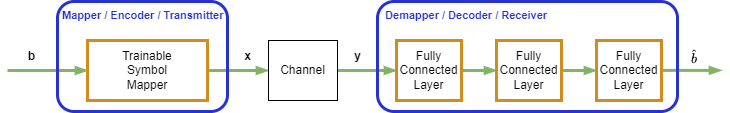During training, you must first pass bits through the encoder, channel, and decoder to obtain the network output. The algorithm then calculates a loss value by comparing the actual output and the expected output. Finally, the algorithm calculates the gradient of the loss function by using the chain rule during backpropagation. The Conventional End-to-End Training of Communications System example shows the design and training of an autoencoder with differentiable channel. If any of the layers, such as the channel layer, is not differentiable, tha back propagation algorithm cannot calculate the gradients for that layer and any layer before that . The model-free training algorithm solves this problem by training the transmitter and receiver separately.

This figure shows the model-free training algorithm. The algorithm first trains the receiver in a loop using the loss calculated at the output of the receiver. Then the algorithm adds a known perturbation to the transmitter output and calculates the transmitter loss based on the receiver loss. The algorithm updates the transmitter weights using the transmitter loss together with estimated gradients. Then the algorithm iterates many times until it achieves a satisfactory loss value. Finally, the algorithm fine-tunes the receiver while keeping the transmitter weights the same. In the following sections, you implement this model-free training algorithm by using custom training loops and custom loss functions.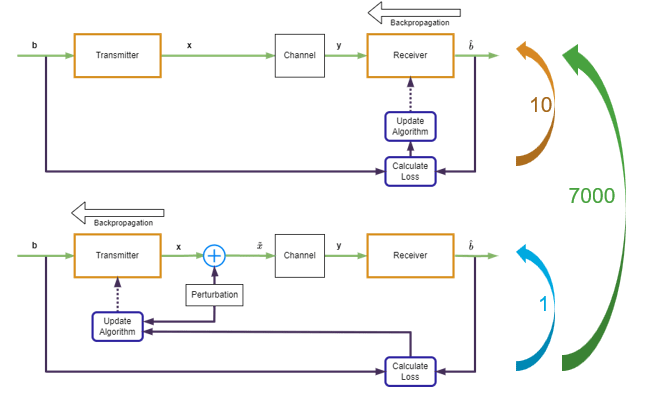#### System Parameters

Design a wireless autoencoder that takes $n$ bits and outputs $n/{N}_{bps}$ complex symbols, where $n$ is the number of coded bits, and ${N}_{bps}$ is the number of bits per symbol. $n$ must be an integer multiple of ${N}_{bps}$. Assume an outer code, such as an LDPC code, with a code rate of $1/2$.

```bitsPerSymbol = 6; % 64-QAM M = 2^bitsPerSymbol; n = 1296; % codeword length codeRate = 1/2;```

#### Training Parameters

Set batch size to 128. Randomly select ${E}_{b}/{N}_{o}$ values between 5 and 8 dB. Set the learning rate to 1e-3.

```B = 128; ebnoMin = 5; ebnoMax = 8; learningRate = 1e-3;```

Convert ${E}_{b}/{N}_{o}$ values to $SNR$ values.

```snrMin = convertSNR(ebnoMin,"ebno", ... BitsPerSymbol=bitsPerSymbol, ... CodingRate=codeRate); snrMax = convertSNR(ebnoMax,"ebno", ... BitsPerSymbol=bitsPerSymbol, ... CodingRate=codeRate);```

### Transmitter Neural Network

The transmitter network takes a bit sequence as an input and maps the bits to complex symbols using the `helperTrainableSymbolMapperLayer` function. The `helperTrainableSymbolMapperLayer` defines constellation points as a learnable property. Set the modulation order to ${2}^{{N}_{bps}}$. To prevent the layer from increasing the output power without a bound as a means to reduce symbol errors and loss, set `UnitAveragePower` to `true` to enforce unit average power for the constellation. The input to the transmitter is a $\left[1×B×n\right]$ array, where $B$ is the batch size. The output of the transmitter is a $\left[2×B×n/{N}_{bps}\right]$ array, where the first dimension is the real and imaginary parts of the complex symbols.

```lgraph = layerGraph([ sequenceInputLayer(1,Name="Bit input",MinLength=n) helperTrainableSymbolMapperLayer(ModulationOrder=2^bitsPerSymbol, ... BitInput=true, ... UnitAveragePower=true, ... Name="mod") ]); txNet = dlnetwork(lgraph); figure plot(txNet)```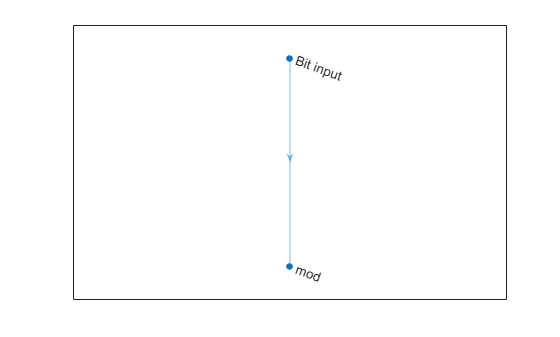The receiver is a fully connected neural network with two hidden layers and an output layer. The input to the receiver is the channel impaired complex symbols in the form of a $\left[1×B×n\right]$ array and $lo{g}_{10}\left({n}_{o}\right)$, which is the per batch channel noise variance array in log domain with size $\left[1×B\right]$. Each hidden layer has 128 outputs followed by ReLU activations. The output layer estimates the LLR values for bits in a symbol, so it has ${N}_{bps}$ outputs.

```lgraph = layerGraph([ sequenceInputLayer(2,Name="rcvd symbols",SplitComplexInputs=true,MinLength=n/bitsPerSymbol) concatenationLayer(1,2,Name="demapper_concat") fullyConnectedLayer(128,Name="demapper_fc1") reluLayer(Name="demapper_relu1") fullyConnectedLayer(128,Name="demapper_fc2") reluLayer(Name="demapper_relu2") fullyConnectedLayer(bitsPerSymbol,Name="demapper_fc3") ]); noInput = sequenceInputLayer(1,Name="no",MinLength=n/bitsPerSymbol); lgraph = addLayers(lgraph,noInput); lgraph = connectLayers(lgraph,"no","demapper_concat/in2"); rxNet = dlnetwork(lgraph); figure plot(rxNet)```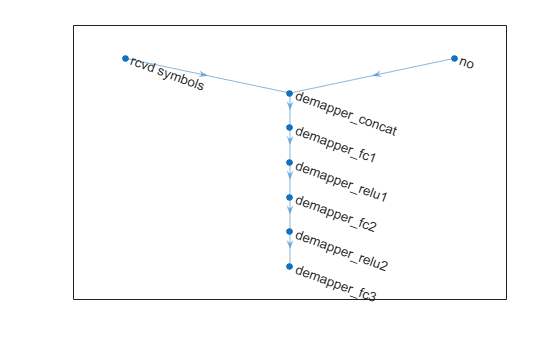### Model-Free Training of Autoencoder

The model-free training algorithm first updates the receiver weights, iterating over the algorithm defined in the Receiver Training section 10 times. Then, the algorithm updates the transmitter weights using the RL-based algorithm described in the Transmitter Training section. The Custom Training Loop section shows the overall training loop that iterates over receiver and transmitter updates.

This figure shows the conventional training process for optimizing the receiver. Pass the binary data, $d$, through the transmitter, channel, and receiver to obtain LLR estimates, $\underset{}{\overset{ˆ}{d}}$. Then calculate the loss value as the binary cross entropy (BCE) between $d$ and $\underset{}{\overset{ˆ}{d}}$ by using the helperBinaryCrossEntropyFromLogits function. Minimizing the BCE is equivalent to maximizing achievable information rate . To obtain gradients and update the receiver weights, use the calculated BCE with the backpropagation algorithm.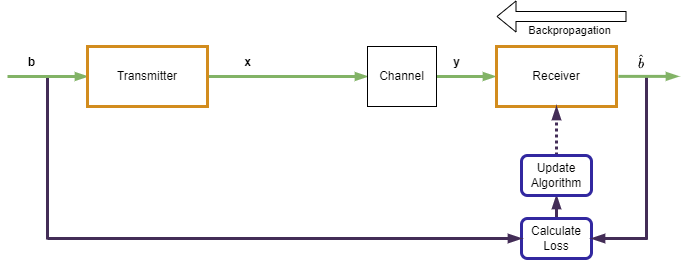Generate random binary input, $d$, and random $SNR$ values for each batch.

```d = dlarray(randi([0 1],1,B,n),'CBT'); snr = rand(1,B,'like',dlarray) ... * (snrMax - snrMin) + snrMin;```

Implement the autoencoder model as a function called `helperAutoencoderRLModel`. The `helperAutoencoderRLModel` function passes the data bits through the transmitter and constructs a complex array by combining the real and imaginary parts. At this point, you can use any channel model function to implement a channel. This example uses a simple AWGN-only channel model to make comparison easy. Even though the AWGN channel is differentiable, this autoencoder does not require a differentiable channel and gradients are not backpropagated from the receiver to the transmitter. The `helperAutoencoderRLModel` function separates the channel-impaired complex symbols into real and imaginary parts and sends them to the receiver network with the noise variance, ${N}_{o}$. The output of the `helperAutoencoderRLModel` function is the LLR estimates of the transmitted bits.

The `helperAutoencoderReceiverModelLoss` function calls the `helperAutoencoderRLModel` function to obtain LLR values. The `helperAutoencoderReceiverModelLoss` function uses LLR estimates, $\underset{}{\overset{ˆ}{d}}$, and transmitted bits, $d$, to calculate the loss for the receiver and performs backpropagation to calculate the gradient estimates. This function also calculates the symbol error rate (`ser`) estimate for the current block of transmitted bits. To enable backpropagation, call the `helperAutoencoderReceiverModelLoss` function through the `dlfeval` (Deep Learning Toolbox) function.

```[lossRxNet,gradientsRx,ser] = dlfeval(@helperAutoencoderReceiverModelLoss,txNet,rxNet,d,snr); lossRxNet```
```lossRxNet = 1x1 single dlarray 0.6921 ```
`ser`
```ser = 1(C) x 128(B) x 1(T) dlarray 0.4020 0.4028 0.4043 0.4005 0.4182 0.4028 0.4190 0.4005 0.3943 0.4012 0.4174 0.4190 0.4213 0.4190 0.4259 0.4005 0.4066 0.4244 0.3989 0.4120 0.4190 0.4290 0.4082 0.3966 0.4182 0.4198 0.4228 0.4128 0.4136 0.4074 0.4128 0.4051 0.4205 0.3997 0.4244 0.4097 0.3981 0.4198 0.4113 0.4306 0.3966 0.3974 0.3966 0.3912 0.4043 0.4074 0.3920 0.4321 0.4221 0.4182 0.4282 0.4074 0.4275 0.4151 0.4113 0.4082 0.4005 0.4128 0.4035 0.4020 0.4136 0.3974 0.3881 0.4390 0.4051 0.3958 0.4105 0.3966 0.4136 0.3804 0.4035 0.3827 0.4028 0.3819 0.3958 0.4035 0.4151 0.4321 0.3912 0.3951 0.3927 0.4182 0.4205 0.4020 0.3827 0.4012 0.4290 0.4105 0.4035 0.4059 0.4290 0.4035 0.4090 0.4043 0.3904 0.4082 0.4074 0.4144 0.3989 0.4460 0.4483 0.3904 0.3943 0.3935 0.4282 0.4082 0.3951 0.4005 0.3897 0.4020 0.4259 0.4074 0.4082 0.3974 0.4136 0.3935 0.4205 0.3850 0.4097 0.3951 0.3935 0.4205 0.4051 0.4028 0.4090 0.4066 0.4012 0.4020 1(C) x 128(B) x 1(T) dlarray ```

Use the Adam algorithm to update the receiver weights by using the `adamupdate` (Deep Learning Toolbox) function. Set the initial value of the average gradients and the average square gradients to an empty array.

```averageGradRx = []; averageSqGradRx = []; iteration = 1; [rxNet,averageGradRx,averageSqGradRx] = ... adamupdate(rxNet,gradientsRx,averageGradRx,averageSqGradRx, ... iteration,learningRate);```

#### Transmitter Training

Assuming that the channel model is not available, train the transmitter using a reinforcement learning (RL) based approach. Apply known perturbations to the transmitter output to enable exploration in the design space. Estimate the gradient of the transmitter weights using an approximate loss function based on the BCE with the `helperPerSymbolBinaryCrossEntropyFromLogits` function. The following figure shows this process.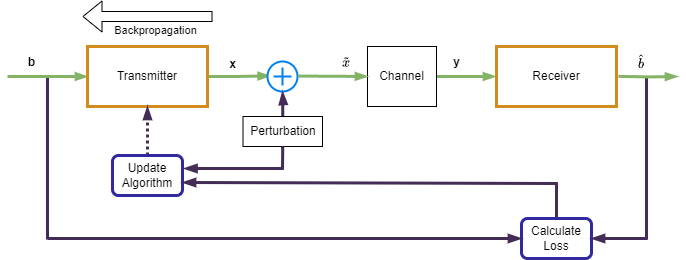The `helperAutoencoderTransmitterModelLoss` function calls the `helperAutoencoderRLModel` function to obtain LLR values. The helperAutoencoderTransmitterModelLoss function uses LLR estimates, $\underset{}{\overset{ˆ}{d}}$, and transmitted bits, $d$, to calculate the approximate loss for the transmitter and performs backpropagation to calculate the gradient estimates. To enable backpropagation, call the helperAutoencoderTransmitterModelLoss function through the `dlfeval` function. Set the variance of the perturbation to 0.01.

```perturbationVar = 0.01; [lossTxNet,gradientsTx] = dlfeval(@helperAutoencoderTransmitterModelLoss,txNet,rxNet,d,snr,perturbationVar);```

Use the Adam algorithm to update the receiver weights. Set the initial value of the average gradients and the average square gradients to empty arrays.

```averageGradTx = []; averageSqGradTx = []; [txNet,averageGradTx,averageSqGradTx] = ... adamupdate(txNet,gradientsTx,averageGradTx,averageSqGradTx, ... iteration,learningRate);```

#### Custom Training Loop

Implement the training algorithm introduced in , which alternates between conventional training of the neural network-based receiver and RL-based training of the transmitter. Perform 7000 iterations of alternating training. Then fine-tune the receiver with 3000 iterations on only the receiver.

```numAlternatingTrainingIterations = 7000; numFinetuningIterations = 3000;```

Check if a GPU is available.

`executionEnvironment = helperCheckGPUDevices();`
```Setting execution environment to 'cpu' ```

On an NVIDIA RTX A5000 GPU with compute capability of 8.6 and 24 GB memory, training takes about an hour, with visualizations turned on. To train the network, set `trainNow` to true. Otherwise, this example loads pretrained networks.

`trainNow =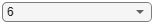false;`

Set up an autoencoder training monitor object to observe loss and SER evolution through iterations.

```if trainNow monitor = helperAutoencoderTrainingMonitor( ... MaxNumIterations=numAlternatingTrainingIterations+numFinetuningIterations, ... UpdatePeriod=10, ... Plots="All", ... ErrorRateSNRRange=convertSNR([6.5 7.0],"ebno",BitsPerSymbol=bitsPerSymbol,CodingRate=codeRate), ... ExecutionEnvironment=executionEnvironment, ... MiniBatchSize=B);```

The Adam algorithm keeps track of average gradients and average square gradients to improve gradient estimates. Define `averageGradTx`, `averageSqGradTx`, `averageGradRx`, and `averageSqGradRx` variables and initialize to empty arrays.

``` averageGradTx = []; averageSqGradTx = []; averageGradRx = []; averageSqGradRx = [];```

To enable autodifferentiation, preallocate the `snr` and `d` arrays as `dlarray` (Deep Learning Toolbox) . If you have a GPU available, define these arrays as `gpuArray` (Parallel Computing Toolbox) and run the training loop on the GPU.

``` if executionEnvironment == "gpu" snr = gpuArray(dlarray(zeros(1,B),"CB")); d = gpuArray(dlarray(zeros(1,B,n,'single'),"CBT")); else snr = dlarray(zeros(1,B),"CB"); d = dlarray(zeros(1,B,n,'single'),"CBT"); end```

The following `for`-loop is the main alternating training loop, which updates the receiver neural network `numReceiverIterations` times, then updates the transmitter network once. This process repeats `numAlternatingTrainingIterations` times.

``` numReceiverIterations = 10; rlTimerStart = tic; for iteration=1:numAlternatingTrainingIterations % Train Rx network rxIteration = 0; while rxIteration < numReceiverIterations snr(:) = rand(1,B) * (snrMax - snrMin) + snrMin; d(:) = randi([0 1],1,B,n); % Evaluate model gradients and loss [lossRxNet,gradientsRx,ser] = dlfeval(@helperAutoencoderReceiverModelLoss,txNet,rxNet,d,snr); % Update network parameters [rxNet,averageGradRx,averageSqGradRx] = ... adamupdate(rxNet,gradientsRx,averageGradRx,averageSqGradRx, ... iteration+rxIteration,learningRate); rxIteration = rxIteration + 1; end % Train Tx network snr(:) = rand(1,B) * (snrMax - snrMin) + snrMin; d(:) = randi([0 1],1,B,n); % Evaluate model gradients and loss [lossTxNet,gradientsTx] = dlfeval(@helperAutoencoderTransmitterModelLoss,txNet,rxNet, ... d,snr,perturbationVar); % Update network parameters [txNet,averageGradTx,averageSqGradTx] = ... adamupdate(txNet,gradientsTx,averageGradTx,averageSqGradTx, ... iteration,learningRate); % Update training monitor constellation = txNet.Layers(2).Constellation; data = [iteration,learningRate,extractdata(gather(lossRxNet)), ... numel(ser), gather(extractdata(ser)), ... size(snr,2), extractdata(gather(snr(1,:,1)))... numel(constellation),constellation]; addData(monitor,data) if monitor.Stop break end end blerEllapsedTime = seconds(toc(rlTimerStart)); blerEllapsedTime.Format = "hh:mm:ss.S" disp('Done alternating training.') disp("BCE: " + gather(extractdata(lossRxNet))) end```

```if trainNow disp("Receiver fine tuning...") for iteration=numAlternatingTrainingIterations+(1:numFinetuningIterations) snr(:) = rand(1,B) * (snrMax - snrMin) + snrMin; d(:) = randi([0 1],1,B,n); % Evaluate model gradients and loss [lossRxNet,gradientsRx,ser] = dlfeval(@helperAutoencoderReceiverModelLoss,txNet,rxNet,d,snr); % Update network parameters [rxNet,averageGradRx,averageSqGradRx] = ... adamupdate(rxNet,gradientsRx,averageGradRx,averageSqGradRx, ... iteration,learningRate); constellation = txNet.Layers(2).Constellation; data = [iteration,learningRate,extractdata(gather(lossRxNet)), ... numel(ser), gather(ser), ... size(snr,2), extractdata(gather(snr(1,:,1)))... numel(constellation),constellation]; addData(monitor,data) if monitor.Stop break end end rlFineTuneEllapsedTime = seconds(toc(rlTimerStart)); rlFineTuneEllapsedTime.Format = "hh:mm:ss.S" if monitor.Stop monitor.Status = "User terminated"; else monitor.Status = "Done"; end else load rl_trained_network_1296 txNet rxNet plot(txNet.Layers(2)) figure imshow("model_free_training_gpu.png") end```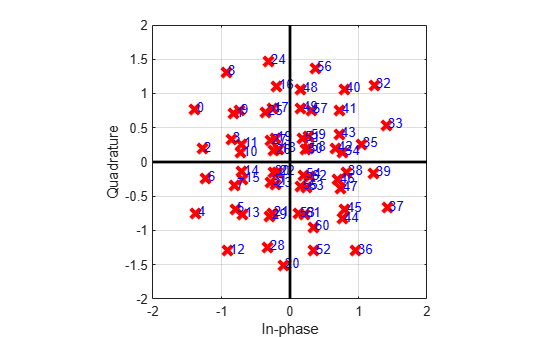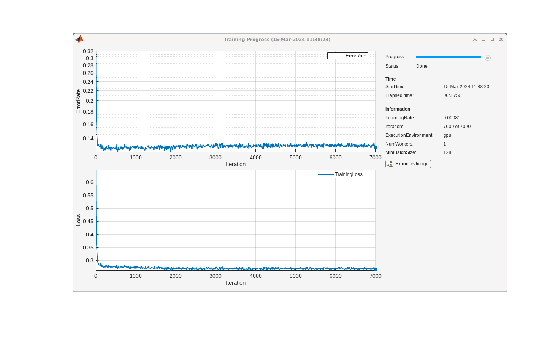### BLER Performance Evaluation

Compare the performance of the model-free trained (RL-based) autoencoder over an AWGN channel to that of a baseline system and a conventionally trained autoencoder, which is trained using the ConventionalEndtoEndTrainingCommunicationsSystemExample script. The baseline system uses 64-QAM modulation with Gray coding. All systems use a (1296,648) LDPC outer code. Increase `targetBlockErrors` and `maxNumBlocks` to increase the accuracy of BLER estimates.

```load conv_trained_network_1296.mat net targetBlockErrors = 200; maxNumBlocks = 4000; blocksPerIteration = 100; ebnoVec = (ebnoMin:0.5:ebnoMax); k=n*codeRate; [cfgLDPCEnc,cfgLDPCDec] = helperLDPCCodeRate12Block1296Info(); maxNumLDPCIter = 10; ber = zeros(length(ebnoVec),3); bler = zeros(length(ebnoVec),3); berUncoded = zeros(length(ebnoVec),3); d = zeros(k,blocksPerIteration,'logical'); blerPlotter = helperBERPlotter( ... "BLER Conventional",'-*b', ... "BLER AI - Conventional",'-or', ... "BLER AI - RL",'-^g', ... "BER Conventional",':*b', ... "BER AI - Conventional",':or', ... "BER AI - RL",':^g'); blerStartTime = tic; disp("Starting BLER simulation...")```
```Starting BLER simulation... ```
```for ebnoIdx = 1:length(ebnoVec) ebno = ebnoVec(ebnoIdx); snr = convertSNR(ebno,"ebno",BitsPerSymbol=bitsPerSymbol,CodingRate=codeRate); snrdl = dlarray(repmat(snr,1,blocksPerIteration,n/bitsPerSymbol),"CBT"); numUncodedErrors = zeros(1,3); numBlockErrors = zeros(1,3); numErrors = zeros(1,3); iteration = 1; while iteration <= maxNumBlocks/blocksPerIteration && all(numBlockErrors < targetBlockErrors) d = randi([0 1],k,blocksPerIteration); c = ldpcEncode(d,cfgLDPCEnc); % Conventional x = qammod(c,M,InputType="bit",UnitAveragePower=true); [y,no] = awgn(x,snr); z = qamdemod(y,M,UnitAveragePower=true,OutputType="llr",NoiseVariance=no); dHat = ldpcDecode(z,cfgLDPCDec,maxNumLDPCIter); err = d ~= dHat; numUncodedErrors(1,1) = numUncodedErrors(1,1) + sum(c~=(z<0),'all'); numBlockErrors(1,1) = numBlockErrors(1,1) + sum(any(err)); numErrors(1,1) = numErrors(1,1) + sum(err,'all'); % AI - Conventional Training cdl = dlarray(permute(c,[3 2 1]),"CBT"); llr = predict(net,cdl,snrdl); z = reshape(permute(extractdata(llr),[1 3 2]),[],blocksPerIteration); dHat = ldpcDecode(-z,cfgLDPCDec,maxNumLDPCIter); err = d ~= dHat; numUncodedErrors(1,2) = numUncodedErrors(1,2) + sum(c~=(z>0),'all'); numBlockErrors(1,2) = numBlockErrors(1,2) + sum(any(err)); numErrors(1,2) = numErrors(1,2) + sum(err,'all'); % AI - RL-Based Training x = predict(txNet,cdl); C = size(x, 1); xC = complex(x(1:C/2, :, :), x(C/2+1:C, :, :)); xCp = permute(stripdims(xC),[3 1 2]); [yCp,no] = awgn(xCp,snr); yC = ipermute(yCp,[3 1 2]); y = dlarray([real(yC); imag(yC)],x.dims()); llr = predict(rxNet,y,dlarray(repmat(no,1,blocksPerIteration,n/bitsPerSymbol),"CBT")); z = reshape(permute(extractdata(llr),[1 3 2]),[],blocksPerIteration); dHat = ldpcDecode(-z,cfgLDPCDec,maxNumLDPCIter); err = d ~= dHat; numUncodedErrors(1,3) = numUncodedErrors(1,3) + sum(c~=(z>0),'all'); numBlockErrors(1,3) = numBlockErrors(1,3) + sum(any(err)); numErrors(1,3) = numErrors(1,3) + sum(err,'all'); iteration = iteration + 1; end bler(ebnoIdx,:) = numBlockErrors / ((iteration-1)*blocksPerIteration); ber(ebnoIdx,:) = numErrors / ((iteration-1)*blocksPerIteration*k); berUncoded(ebnoIdx,:) = numUncodedErrors / ((iteration-1)*blocksPerIteration*n); blerEllapsedTime = seconds(toc(blerStartTime)); blerEllapsedTime.Format = "hh:mm:ss.S"; disp(string(blerEllapsedTime) + " - Eb/No = " + ebno + "dB") addpoints(blerPlotter,ebno,bler(ebnoIdx,1),bler(ebnoIdx,2),bler(ebnoIdx,3), ... berUncoded(ebnoIdx,1),berUncoded(ebnoIdx,2),berUncoded(ebnoIdx,3)); end```
```00:00:03.4 - Eb/No = 5dB 00:00:05.7 - Eb/No = 5.5dB 00:00:08.5 - Eb/No = 6dB 00:00:12.2 - Eb/No = 6.5dB 00:00:20.2 - Eb/No = 7dB 00:00:41.8 - Eb/No = 7.5dB 00:01:07.8 - Eb/No = 8dB ```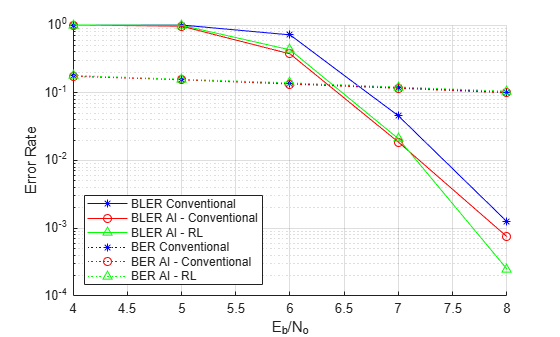BLER curves show that the conventional autoencoder, which has full knowledge of the differentiable channel, outperforms the baseline system by about 0.7dB at 10% BLER. The RL-based autoencoder, which does not have the channel model, performs within 0.1 dB of the conventional autoencoder.

### Discussions and Further Exploration

In this example, you implement a complex AI-based physical layer that uses custom training loops and custom loss functions. You simulate the system BLER performance over a link with a conventional LDPC outer code. To explore the system performance further, replace the channel model with more complex models such as `comm.RayleighChannel`, `comm.RicianChannel,` and `comm.RayTracingChannel`. Alternatively, use standards-based channels such as `nrCDLChannel`, `nrTDLChannel`, and `nrHSTChannel`. Vary the number of bits per symbol, `bitsPerSymbol`, and block size, $n$.

The ConventionalEndtoEndTrainingCommunicationsSystemExample script shows how to train the same network with a known channel model and back propagation.

### References

 F. Ait Aoudia and J. Hoydis, “Model-Free Training of End-to-End Communication Systems,” in IEEE Journal on Selected Areas in Communications, vol. 37, no. 11, pp. 2503-2516, Nov. 2019, doi: 10.1109/JSAC.2019.2933891.

 S. Cammerer, F. A. Aoudia, S. Dörner, M. Stark, J. Hoydis and S. ten Brink, "Trainable Communication Systems: Concepts and Prototype," in IEEE Transactions on Communications, vol. 68, no. 9, pp. 5489-5503, Sept. 2020, doi: 10.1109/TCOMM.2020.3002915.